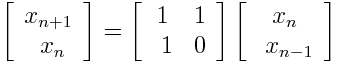# Power method and Fibonacci numbers

Take an n × n matrix A and a vector x of length n. Now multiply x by A, then multiply the result by A, over and over again. The sequence of vectors generated by this process will converge to an eigenvector of A. (An eigenvector is a vector whose direction is unchanged when multiplied by A. Multiplying by A may stretch or shrink the vector, but it doesn’t rotate it at all. The amount of stretching is call the corresponding eigenvalue.)

The eigenvector produced by this process is the eigenvector corresponding to the largest eigenvalue of A, largest in absolute value. This assumes A has a unique eigenvector associated with its largest eigenvalue. It also assumes you’re not spectacularly unlucky in your choice of vector to start with.

Assume your starting vector x has some component in the direction of the v, the eigenvector corresponding to the largest eigenvalue. (The vectors that don’t have such a component lie in an n-1 dimensional subspace, which would has measure zero. So if you pick a starting vector at random, with probability 1 it will have some component in the direction we’re after. That’s what I meant when I said you can’t start with a spectacularly unlucky initial choice.) Each time you multiply by A, the component in the direction of v gets stretched more than the components orthogonal to v. After enough iterations, the component in the direction of v dominates the other components.

What does this have to do with Fibonacci numbers? The next number in the Fibonacci sequence is the sum of the previous two. In matrix form this saysThe ratio of consecutive Fibonacci numbers converges to the golden ratio φ because φ is the largest eigenvalue of the matrix above.

The first two Fibonacci numbers are 1 and 1, so the Fibonacci sequence corresponds to repeatedly multiplying by the matrix above, starting with the initial vector x = [1 1]T. But you could start with any other vector and the ratio of consecutive terms would converge to the golden ratio, provided you don’t start with a vector orthogonal to [1 φ]T. Starting with any pair of integers, unless both are zero, is enough to avoid this condition, since φ is irrational.

We could generalize this approach to look at other sequences defined by a recurrence relation. For example, we could look at the “Tribonacci” numbers. The Tribonacci sequence starts out 1, 1, 2, and then each successive term is the sum of the three previous terms. We can find the limiting ratio of Tribonacci numbers by finding the largest eigenvalue of the matrix below.This eigenvalue is the largest root of x3x2x – 1 = 0, which is about 1.8393. As before, the starting values hardly matter. Start with any three integers, at least one of them non-zero, and define each successive term to be the sum of the previous three terms. The ratio of consecutive terms in this series will converge to 1.8393.

By the way, you could compute the limiting ratio of Tribonacci numbers with the following bit of Python code:

      from scipy import matrix, linalg
M = matrix([[1, 1, 1], [1, 0, 0], [0, 1, 0]])
print( linalg.eig(M) )


Update: The next post generalizes this one to n-Fibonacci numbers.

## 2 thoughts on “Power method and Fibonacci numbers”

1. I left out some details to simplify the exposition above. For example, I used “convergence” loosely. To get a convergent sequence, you’d need to normalize the vectors. Otherwise the length of the vectors might go to zero or diverge to infinity.